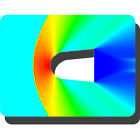Aerodynamics Tool

All Android applications categories

All Android games categories# Aerodynamics Tool

2 10

10 Users
rating

## Screenshots

Description

The app is handy tool for Aerodynamic Engineer and Academics. It contains Isentropic Relations, Normal Shock Relations, Oblique-Shock (Theta-Beta-Mach) Relations, Prandtl-Meyer Expansion wave Relations, Pitot Tube Velocity computations, Y Plus or Y+ calculations.

1. Basic Aerodynamic Calculator- Calculation of Dynamic Pressure, Reynolds Number, Force and Moment Coefficient for Incompressible and Compressible flow.

2. Isentropic Relations - If any one of the variables, M, Po/P, To/T, Rho/Rh or A/A* is known the remaining variable values can be computed for any specific heat ratio (Gamma).

3. Normal Shock- Select any known variable from list, M1, P2/P1, T2/T1, M2, rho2/rho1, Po2/Po1, and Po2/P1 and user can calculate remaining variable values for any specific heat ratio (Gamma).. Indices 1 and 2 are for before shock and after shock respectively.

4. Oblique Shock - Theta-Beta-Mech number relation. If values of any two variable known then user can calculate value of remaining one for any specific heat ratio (Gamma)..

5. Vortex Lattice Method - Vortex Lattice Method is a method used to calculate lift coefficient of Finite Wing at low speed. It gives fairly good CL estimation for Swept wing compared to Vortex Panel Method. This tool is helpful for primary aerodynamic design iterations. The method presented here uses 'n' spanwise divisions and one chordwise division of wing. The final output is slope of CL vs Alpha curve and it is CL per degree angle of attack.

6. Prandtl-Meyer expansion wave relations - Compute v1 from M1 (Mach Number before expansion wave). v is Prandtl-Meyer function. Add deflection angle theta to v1 to get v2. From v2 compute M2 (Mach Number after expansion wave) and all this for any specific heat ratio (Gamma).

7. Pitot Tube - Divided in two categories. One for Incompressible Flow and Other for Compressible Flow. In case of Incompressible flow, user can input height of Pitot Tube column in Meters of Water, Mercury or Any known fluid to calculate free stream velocity. The compressible flow case takes inputs for Total Pressure and Static Pressure measured by Pitot Tube and calculates Free Stream Mach Number. User need not to worry about Subsonic or Supersonic flows. These are identified and solved accordingly.

8. Y Plus or Y+ - It calculates first cell height of grid near wall to resolve boundary layer.

9. Check points for Inputs to avoid wrong inputs and flash suggestions if any.

If found any Bug or wrong output, please mail me regarding issue...Thanks in advance

from 2 reviews

"Awesome"

10